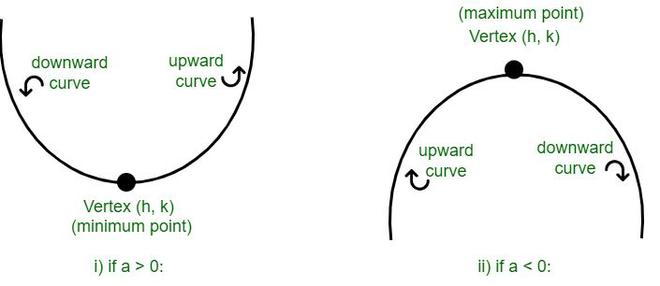# How to find the turning point of a parabola?

• Difficulty Level : Hard
• Last Updated : 02 Jun, 2022

When a quadratic equation is represented graphically with a U – shape, it is called a parabola. A parabola can also be defined as a plain curve where any point on that curve is equidistant from a fixed point, the focus.  The turning point of any curve or parabola is the point at which its direction changes from upward to downward or vice-versa. The turning point of a parabola is called the vertex. The standard form of the parabola is y = ax2 + bx + c. The vertex form of the parabola with Vertex (h, k) is y = a(x – h)2 + k.### Turning point of the parabola

To obtain the turning point or vertex (h, k) of the parabola, we can transform this equation to the vertex form of the parabola: y = a(x – h)2 + k. We can perform this by using the “Completing the Squares” method.

• Subtract c from LHS and RHS.

y – c = ax2 + bx

• Take “a” as a  common factor on the RHS.

y – c = a(x2 + (b/a)x)

• Add the term a(b / 2a)2 to both RHS and LHS.

y – c + (b2/4a) = a(x2 + (b/a)x + (b /2a)2)

• Now the equation on RHS is of the form (m + n)2.

y – (c + (b2/4a)) = a(x + (b/2a)2)

By comparing this equation with the vertex form of the parabola, we can observe the following relation between the values of a, b, c, and h, k.

Vertex (h, k) = (-b/2a, c – (b2/4a))

### Sample Problems

Problem 1: Find the turning point of a parabola defined by the equation y = 5x2 + 3x + 2.

Solution:

Given, y = 5x2 + 3x + 2: a = 5 b = 3 c = 2

Using the formula mentioned above, (h, k) = (-b/2a, c – (b2/4a))

⇒ h = -3/(2 × 5) = -3/10 = 0.3

⇒ k = 2 – (3 × 3)/(4 × 5) = 2 – (9/20) = 1.55

So, (h, k) = (0.3, 1.55)

Problem 2: Given the function F(x) = 7x2 + 5x + 8, find the value of x for which it is increasing.

Solution:

The equation, 7x2 + 5x + 8 is in the form of a parabola.

Where, a = 7, b = 5 and c = 8

We know that for a > 0, the parabola has an upward or increasing direction for x > h.

Using the formula mentioned above, (h, k) = (-b/2a, c – (b2/4a))

⇒ h = -5/(2 × 7) = -5/14 = -0.357

So, the function is increasing for (x > -0.357)

Problem 3: What is the minimum value of the function y = 3x2 + 8x + 1?

Solution:

The equation y = 3x2 + 8x + 1 is of the form of a parabola.

Where, a = 3, b = 8 and c = 1

We know that for a > 0, the parabola has its minimum value at its Vertex.

Using the formula mentioned above, Vertex V (h, k) = (-b/2a, c – (b2/4a))

⇒ h = -8/(2 × 3) = -8/6 = -1.33

⇒ k = 1 – (8 × 8/(4 × 3)) = 1 – (64/12) = -4.33

Therefore, the minimum value of the function is at (-1.33, -4.33).

Problem 4: Find the turning point of a parabola defined by the equation y = 1x2 + 2x + 3.

Solution:

Given, y = 1x2 + 2x + 3,

a = 1

b = 2

c = 3

Using the formula mentioned above, the turning point or the vertex is,

(h, k) = (-b/2a, c – (b2/4a))

⇒ h = -2/(2 × 1) = -1

⇒ k = 3 – (2 × 2/4) = 2

So, (h, k) = (-1, 2)

Problem 5: Given the function F(x) = -2x2 + 2x + 1, find the value of x for which it is decreasing.

Solution:

The equation, -2x2 + 2x + 1 is of the form of a parabola.

Where, a = -2, b = 2 and c = 1

We know that for a < 0, the parabola has a downward or decreasing direction for x > h.

Using the formula mentioned above,

(h, k) = (-b/2a, c – (b2/4a))

⇒ h = -2/(2 × (-2)) = 1/2 = 0.5

So, the function is increasing for (x > 0.5).

Problem 6: What is the vertex of the parabola y = -8x2 + 8x + 1.

Solution:

Given y = -8x2 + 8x + 1:

a = -8

b = 8

c = 1

Using the formula mentioned above,(h, k) = (-b/2a, c – (b2/4a))

⇒ h = -8/(2 × (-8)) = 1/2 = 0.5

⇒ k = 1 – (8 × 8/(4 × (-8))) = 1 + (64/32) = 3

So, (h, k) = (0.5, 3)

Problem 7: Given the function y = -9x2 + 2x + 5, find the maximum point of the curve.

Solution:

The equation y = -9x2 + 2x + 5 is of the form of a parabola.

Where, a = -9, b = 2 and c = 5

We know that for a < 0, the maximum point of the parabola is at (h, k).

Using the formula mentioned above,(h, k) = (-b/2a, c – (b2/4a))

⇒ h = -2/(2 × (-9)) = 1/9 = 0.11

So, the function is increasing for (x > 0.5)

My Personal Notes arrow_drop_up